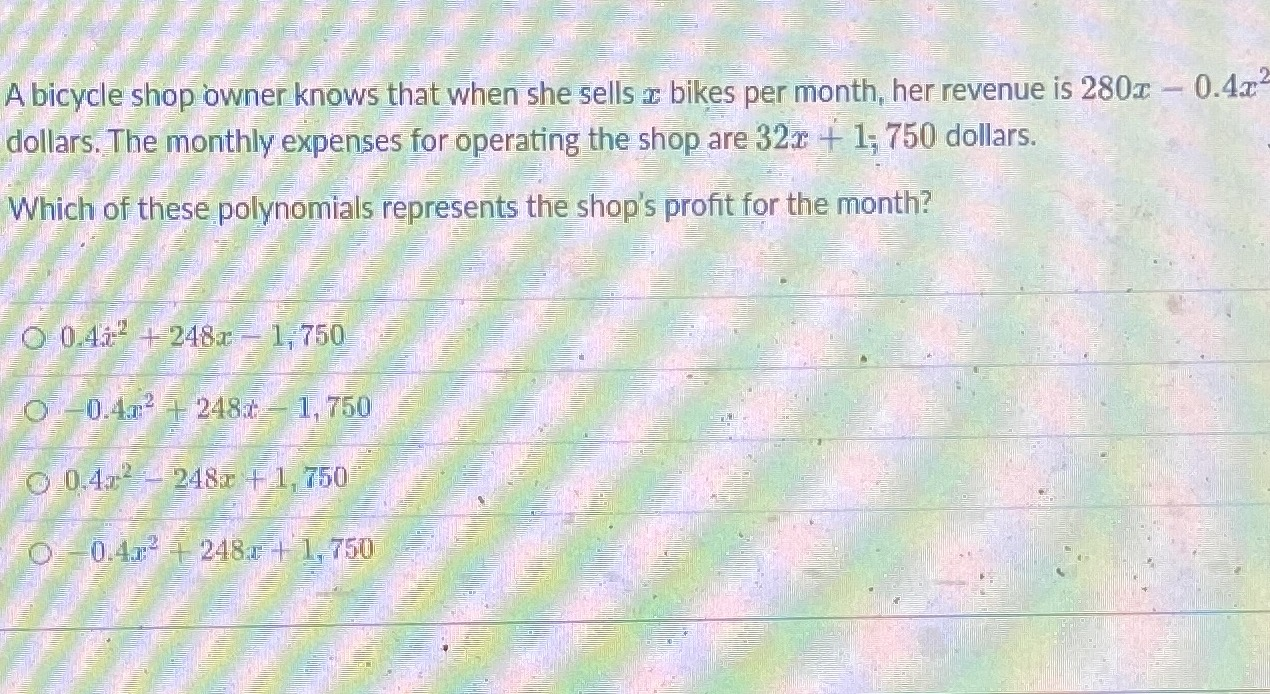### ¿Todavía tienes preguntas de matemáticas?

Pregunte a nuestros tutores expertos
Algebra
PreguntaA bicycle shop owner knows that when she sells $$x$$ bikes per month, her revenue i$$280 x - 0.4 x ^ { 2 }$$ dollars. The monthly expenses for operating the shop are $$32 x + 1 ; 750$$ dollars. Which of these polynomials represents the shop's profit for the month?

$$0.4 x ^ { 2 } + 248 x - 1,750$$

$$0 - 0.4 x ^ { 2 } + 248 x - 1,750$$

$$0.4 x ^ { 2 } - 248 x + 1,750$$

$$0 - 0.4 x ^ { 2 } + 248 x + 1,750$$